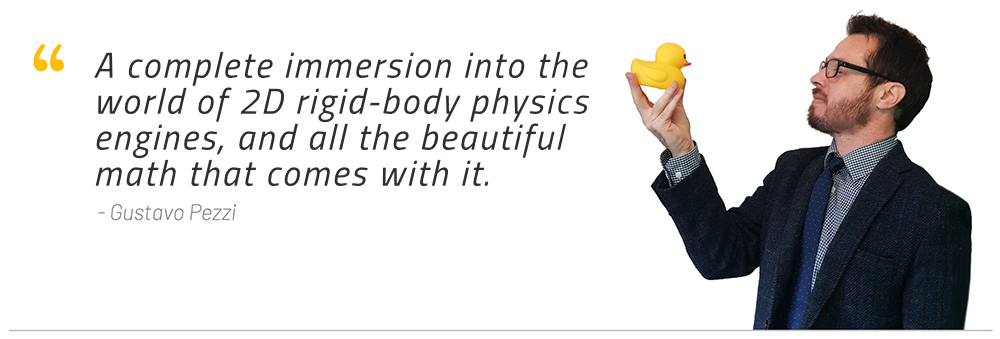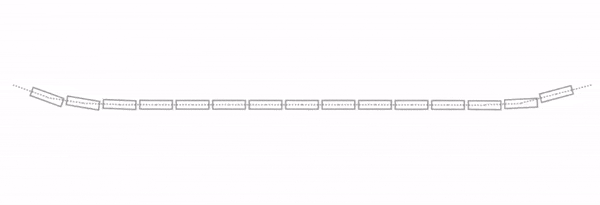This course includes:

(updated Oct 2021)

• 35 hours of on-demand video

• Access on mobile and desktop

• Certificate of completion

• 14-day money back guarantee

• Secure checkout through Credit Card or PayPal

Is this course for you?

This course is a complete immersion into the world of 2D game physics. You'll learn all the required math, going from basic vector math, to matrices, until we touch some calculus to solve physics constraints. This course is aimed at students that already know some programming, but want to learn how a simple 2D physics engine (like Box2D or Chipmunk2D) work under the hood.Prerequisites

Before taking this course, you must have:

• A basic knowledge of coding (variables, if-else, loops, functions, OOP)

• Some experience with either Linux, Windows, or macOS

Particle Physics

We'll begin our journey looking at simple particle physics. We'll start by learning what simulation via software really is, how it works, review the required math, and look at the fundamentals of movement.Rigid-Body Physics

Once we get the basics out of the way, we'll start looking at rigid-body physics. This means we'll learn about different shapes, handling angular movement, and also looking at collision detection and collision resolution. This is a super important topic and we'll have some fun poking rigid-bodies around.Constraints

We'll also take a deep look at constrained rigid-body simulation, which will help us add some different types of objects to our engine (like joints, anchor points, and ragdolls). Ultimately, constraints will help improve the stability of our engine, and they are an opportunity for us to look at some interesting math topics, including a little bit of calculus.Discuss course topics and engage with other students using the discussion board

• Money back guarantee

We offer a 14-day money back guarantee for all our courses. No questions asked.

Course Content

• 1
• 2
• 3
Vector Operations
• Vector Magnitude
• Methods for Vector Addition & Subtraction
• Vector Equality
• Scaling Vectors
• Static Methods
• Applications of Vector Addition & Subtraction
• Quiz: Vector Arithmetic
• 4
Dot Product & Cross Product
• Dot Product
• Is the Dot Product Commutative?
• Dot Product Length
• Quiz: Dot Product
• Cross Product
• Coding the Cross Product Method
• Exercise: Perpendicular 2D Vector
• Perpendicular 2D Vector
• Exercise: Vec3 Methods
• Vec3 Methods
• Applications of Dot Product & Cross Product
• Quiz: Cross Product
• 5
Normalizing Vectors
• Vector Normalization
• Coding the Normalization Method
• 6
Vector Transformations
• Scaling, Translating, and Rotating Vectors
• Quick Review of Sine & Cosine
• Vector Rotation Proof (x-component)
• Vector Rotation Proof (y-component)
• Coding the Vec2 Rotate Method
• Concluding our JavaScript Vector Class
• 7
Vectors in C++
• A Quick Look at C++ Vec2 Syntax
• 8
Starting our C++ Project
• Technologies & Dependencies
• Folder Structure
• Initial Project Files
• Compiling using GCC & Linux
• Makefile
• Configuring Visual Studio on Windows
• 9
Particle Physics
• Introduction to Particle Physics
• Particle Class
• Particle Velocity
• Exercise: Operator + to Add Vectors
• Using the + Operator to Add Vectors
• Controlling our Framerate
• Framerate Independent Movement
• Clamping Invalid DeltaTime Values
• Quiz: Framerate & DeltaTime
• Moving in a Constant Velocity
• Changing the Particle's Velocity
• Keeping the Particle Inside the Window
• 10
Simulating Movement
• Constant Acceleration
• Discrete vs. Continuous
• Integration & Movement Simulation
• Different Notations for Differentiation
• Integral as Continuous Sum
• Different Integration Methods
• Particle Integrate Function
• Quiz: Integration
• 11
Applying Forces
• Applying Forces to Particles
• Kinematics vs. Kinetics
• Particles with Different Mass
• The Weight Force
• Inverse of the Mass
• Quiz: Forces & Mass
• Applying Forces with the Keyboard
• 12
Drag & Friction Forces
• Drag Force
• Drag Force Function
• Handling Mouse Clicks with SDL
• Unexpected Drag Behavior
• Friction Force
• Friction Force Function
• Examples of Forces in Games
• 13
Gravitational Attraction
• Gravitational Attraction Force
• Gravitational Attraction Force Function
• 14
Spring Forces
• Spring Force
• Spring Force Function
• Exercise: Spring Forces
• Multiple Particles: Chain
• Multiple Particles: Soft body
• Soft Bodies & Verlet Integration
• 15
Rigid-Body Physics
• Rigid-Bodies
• Shapes
• Shape Class
• Shape Class Implementation
• Circle Shape
• Angular Velocity & Angular Acceleration
• Torque & Moment of Inertia
• Different Shapes & Moment of Inertia
• Circle Shape Angular Motion
• Angular Velocity DIrection
• Quiz: Angular Motion
• 16
Shapes & Vertices
• Box Vertices
• Local Space vs. World Space
• Body Update Function
• Why Not a Shape Draw Function?
• No Draw Method in the Shape Class
• 17
Colliding Circles
• Circle-Circle Collision Detection
• Circle-Circle Collision Class
• Circle-Circle Collision Implementation
• Collision Contact Information
• Correctly Displaying Colliding Bodies
• Collision Information Code
• Broad Phase & Narrow Phase
• The Projection Method
• Objects with Infinite Mass
• Floating-Point Comparison
• 18
Impulse & Momentum
• Impulse Method & Momentum
• Impulse
• Deriving the Linear Impulse Formula
• Simplifying the Impulse Method Formula
• Coding the Linear Impulse Method
• Is Linear Collision Response Enough?
• Quiz: Collisions
• 19
Colliding Boxes
• AABB Collision Detection
• SAT: Separating Axis Theorem
• Different SAT Approaches
• Finding Minimum Separation with SAT
• Polygon-Polygon Collision Code
• Code to Find SAT Minimum Separation
• Refactoring the SAT Separation Function
• Support Points?
• Finding Extra Collision Information with SAT
• Polygon-Polygon Collision Information
• 20
Angular Collision Resolution
• Linear & Angular Velocity At Point
• Post-Collision Velocity At Point
• Computing Linear & Angular Impulse
• Collision Distance Vectors Ra-Rb
• 2D Cross Product Simplification
• Coding the Impulse Along Normal
• Exercise: Impulse Along Tangent
• Friction Impulse Along Tangent
• Removing Window Boundaries Check
• Collision Resolution in 3D
• 21
Colliding Circles & Polygons
• Circle-Polygon Collision Detection
• Finding Polygon's Nearest Edge with Circle
• Exercise: Circle-Polygon Edge Regions
• Circle-Polygon Collision Information
• Circle-Polygon Collision Resolution
• Exercise: Polygons with Multiple Vertices
• Polygon with Multiple Vertices
• 22
Rendering Textures
• Rendering Circle Texture
• Textures Inside Rigid-Bodies?
• 23
The World Object
• World Class
• Implementing World Functions
• 24
Collision Instability
• Refactoring Function to Update Vertices
• Local Solvers vs. Global Solvers
• A Naive Iterative Positional Correction
• 25
Constraints
• Constrained Rigid-Body Physics
• Position vs. Velocity Constraints
• Example Velocity Constraint & Bias Factor
• Example Distance Constraint & Bias Factor
• Constraint Forces & Constrained Movement
• Force-Based vs. Impulse-Based Constraints
• The Constraint Class
• 26
Matrices
• VecN Class
• Implementing VecN Functions
• Matrices
• MatMN Class
• Matrix Transpose
• Matrix Multiplication
• Matrix Multiplication Function
• Seeing Beyond the Matrix
• 27
Distance Constraint
• Generalized Velocity Constraint
• Solving Violated Velocity Constraints
• Constraint Class Inheritance
• Distance Constraint
• Joint Constraint Class
• Joint Constraint vs. Distance Constraint
• Converting World Space to Local Space
• Inverse Rotation Matrix
• World List of Constraints
• Refactoring Body Update
• Deriving the Distance Jacobian
• Populating the Distance Jacobian
• Solving System of Equations (Ax=b)
• Gauss-Seidel Method
• Constrained Pendulum
• Quiz: Distance Constraints
• 28
System of Constraints
• Solving System of Constraints Iteratively
• Warm Starting
• Exercise: Ragdoll
• Ragdoll with Joint Constraints
• Preventing NaN Errors
• 29
Penetration Constraint
• Penetration Constraint
• Deriving the Penetration Jacobian
• Penetration Constraint Class
• Solving Penetration Constraints
• Linear Complementary Problem
• Penetration Warm Starting
• A Quick Note on Penetration Warm Starting
• Penetration Constraint Friction
• Clamping Friction Magnitude Values
• Penetration Constraint Bounciness
• 30
Stack of Boxes
• Unstable Stack of Boxes
• Allowing for Multiple Contact Points
• Reference & Incident Edges
• Finding Incident Edge
• Clipping Function
• Testing Multi-Contact Boxes
• Testing Multiple Objects & Constraints
• 31
Optimization Techniques
• Contact Caching
• Continuous Collision Detection
• Computing the Moment of Inertia of Polygons
• 32
Conclusion
• Conclusion & Next Steps
• Moving Forward

Instructor

•Gustavo Pezzi

Gustavo teaches computer science and mathematics at BPP University, London. He studies how teaching game programming can help enhance awareness and understanding of basic mathematics and physics. He is also a professional programmer with several years of experience in the computing industry. His academic path includes institutions such as Pittsburg State University, City University of London, and University of Oxford.